DDG（离散微分几何）：对微分几何快速粗暴的一个介绍 | HyperPlane

## 曲面的几何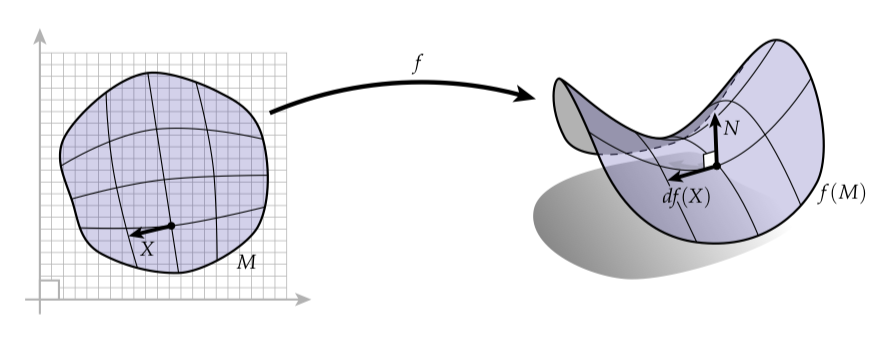$\sqrt{df(X) \cdot df(X)}$

$\mathsf{g}(X, \Upsilon) = df(X) \cdot df(\Upsilon)$

$df(X) \cdot u = 0$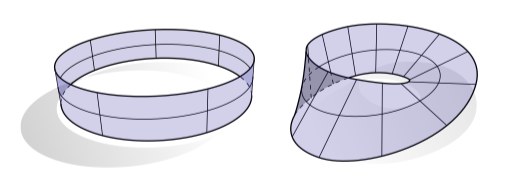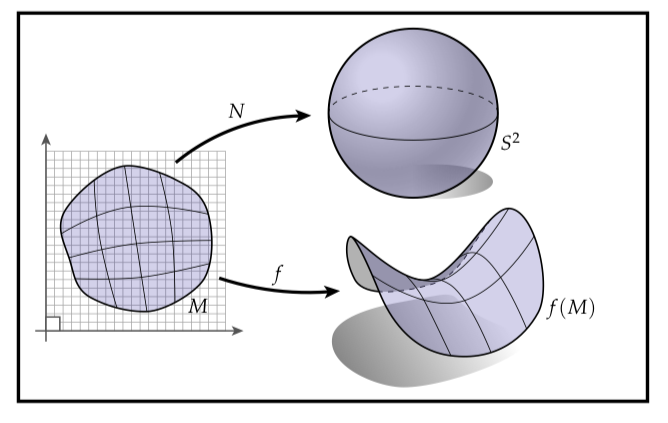### 共形坐标系

$|df(X)| = |X|$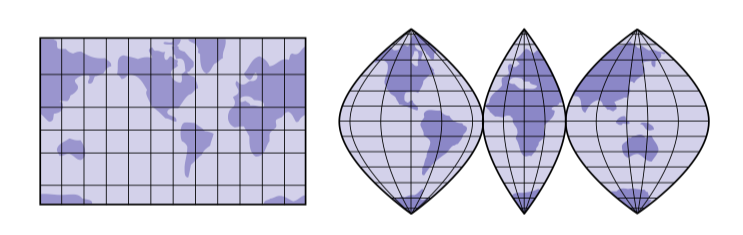$df(X) \cdot df(\Upsilon) = a\langle X, \Upsilon \rangle$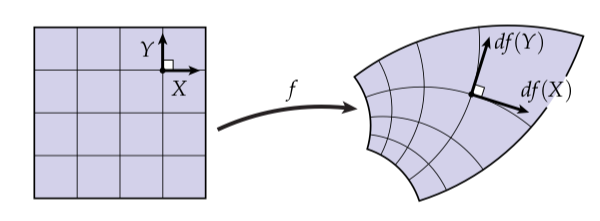## 导数和正切向量

### 在实线上的导数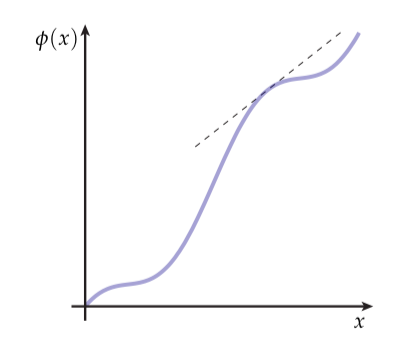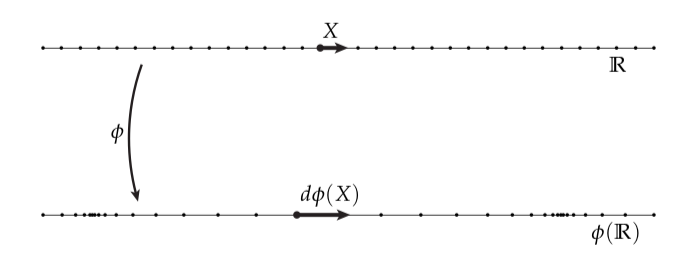$\phi' = d \phi (X)$

### 方向导数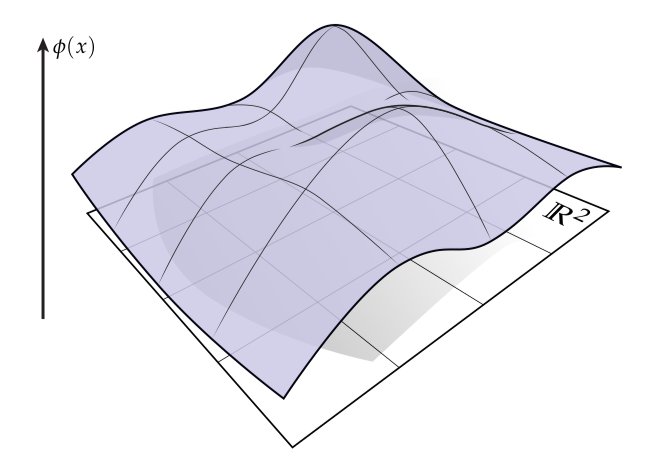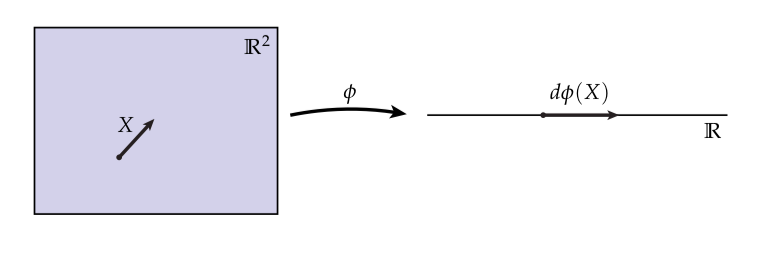## 曲线的几何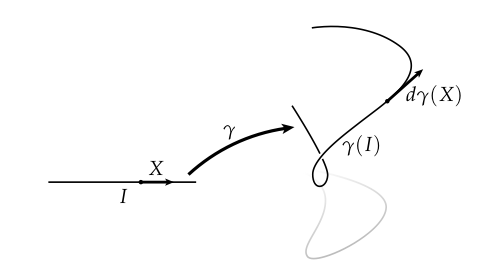$|d\gamma(X)| = \sqrt{d\gamma(X) \cdot d\gamma(X)}$

$|d\gamma(X)| = |X|$

### 曲线的曲率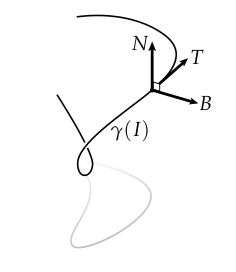$T = d\gamma(X)$

$\mathbb{R}^3$中与曲线相切的单位向量。将这个想法向前推进一步，我们可以看到当我们沿着$\gamma$移动时切向的变化。由于$T$可能按照任何比例变化（也可能完全不变！），我们将这个变化分离为两个部分：一个被成为主法线（principal normal）的单位向量$N$表示变化的方向，和一个被成为曲率（curvature）的标量$\kappa \in \mathbb{R}$表示变化的程度：

$dT(X) = - \kappa N$

$\underbrace{\begin{bmatrix} T' \\ N' \\ B' \end{bmatrix}} _{Q' \in \mathbb{R}^3}= \underbrace{ \begin{bmatrix} 0 & -\kappa & 0 \\ \kappa & 0 & -\tau \\ 0 & \tau & 0 \end{bmatrix} }_{A \in \mathbb{R}^{3 \times 3}} \underbrace{ \begin{bmatrix} T \\ N \\ B \end{bmatrix} }_{Q \in \mathbb{R}^3}$

### 可视化曲线

$\mathcal{S}$曲率是什么？当$\mathcal{S}$有半径$r$时，在单位速度下需要花费$2 \pi r$的时间去绕这个圆一圈。在这段时间中，切线方向转了$2 \pi$的角度。当然，由于$T$有单位长度，$T$上瞬间的变化仅仅通过角度的瞬间变化描述。所以我们最终得到（第三个等号是总变化/时间）

$\kappa = |\kappa N| = |dT(X)| = 2 \pi /2 \pi r = 1 / r$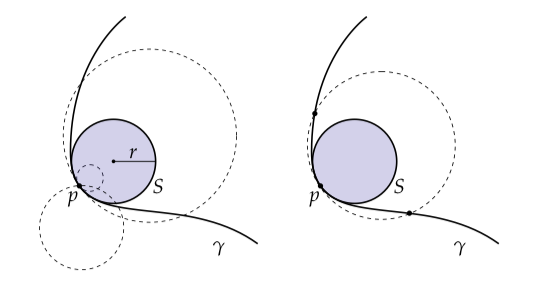## 曲面的曲率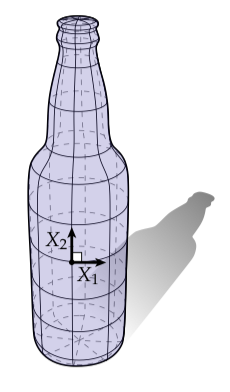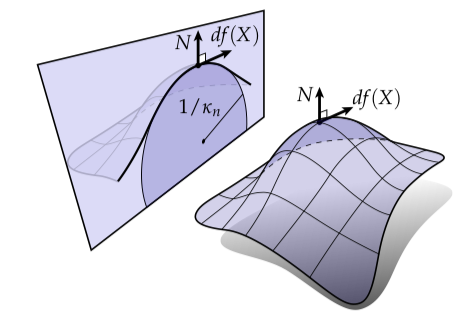$\kappa_n(X) = \frac{df(X) \cdot dN(X)}{|df(X)|^2}$

### 主曲率、平均曲率和高斯曲率$df(SX) = dN(X)$

$\mathcal{S}X_i = \kappa_i X_i$

$\kappa_n(\Upsilon) = \kappa_1 \cos^2\theta + \kappa_2 \sin^2\theta$

$H = \frac{\kappa_1 + \kappa_2}{2}$

$K = \kappa_1 \kappa_2$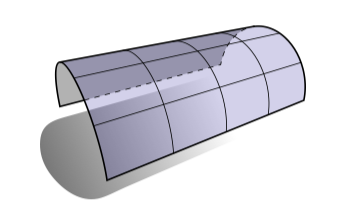0平均曲率的曲面被称为最小曲面（minimal surfaces），因为（就像我们稍后将看到的）它们最小化曲面空间（在确定的约束下）。最小曲面趋向鞍状，因为主曲率有相同的大小但是符号相反：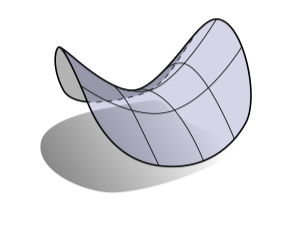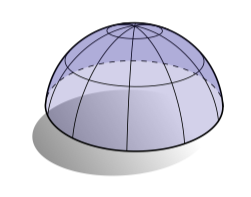### 基本型

$I(X, \Upsilon) \coloneqq \mathsf{g}(X, \Upsilon)$

$II(X,\Upsilon) \coloneqq -\mathsf{g}(\mathcal{S}X, \Upsilon) = -dN(X) \cdot df(\Upsilon)$

## 坐标系中的几何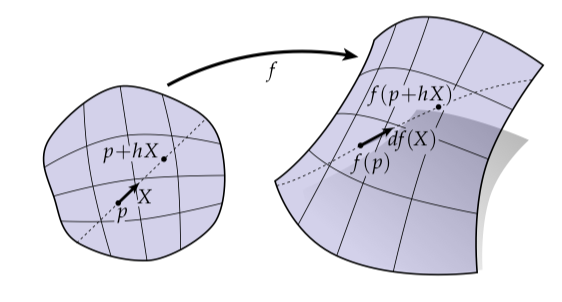$df_p(X) = \lim_{h \rightarrow 0} \frac{f(p + hX) - f(p)}{h}$

$\mathsf{J} = \begin{bmatrix} \partial f^1/ \partial x^1 & \partial f^1/ \partial x^2 \\ \partial f^2/ \partial x^1 & \partial f^2/ \partial x^2 \\ \partial f^3/ \partial x^1 & \partial f^3/ \partial x^2 \end{bmatrix}$

$f(x^1, x^2) = (f_1(x^1,x^2), f_2(x^1,x^2), f_3(x^1,x^2))$

### 被认为有害的坐标系表示

$\begin{bmatrix} 0 & 1 \\ 1 & 0 \end{bmatrix}$

$f:\mathbb{R}^2 \rightarrow \mathbb{R}^2;u \mapsto f(u)$

$\mathsf{g} : \mathbb{R}^2 \times \mathbb{R}^2 \rightarrow \mathbb{R};(u,v) \mapsto \mathsf{g}(u,v)$

$f(u) = \mathsf{Au}$

$\mathsf{g}(u,v) = \mathsf{u^TBv}$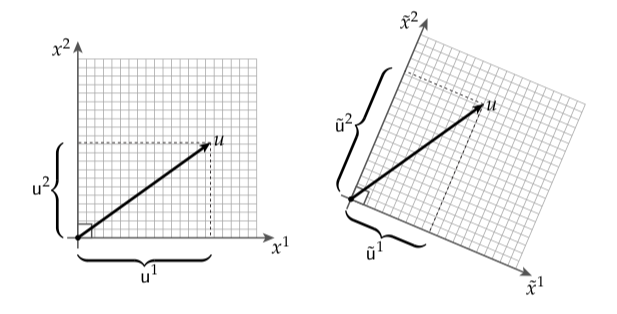\begin{aligned} \mathsf{\tilde{u}} &= \mathsf{Pu} \\ \mathsf{\tilde{v}} &= \mathsf{Pv} \end{aligned}

$f(u) = \mathsf{PAu} = \mathsf{PAP}^{-1}\mathsf{\tilde{u}}$

$\mathsf{g}(u,v) = \mathsf{u^TBv} = \mathsf{(P^{-1}\tilde{u})^T B (P^{-1}\tilde{v})} = \mathsf{\tilde{u}^T (P^{-T}BP^{-T}) \tilde{v}}$

$\mathsf{A \mapsto PAP^{-1}}$

$\mathsf{B \mapsto P^{-T} B P^{-T}}$

### 曲面几何中的标准矩阵

$\mathsf{g}(u,v) = df(u) \cdot df(v)$

$\mathsf{u^T} I \mathsf{v = (Ju)^T (Jv)}$

$I = \mathsf{J^TJ}$

$I = \begin{bmatrix} \mathsf{E} & \mathsf{F} \\ \mathsf{F} & \mathsf{G} \end{bmatrix}$

（能明确为什么“$\mathsf{F}$”出现两次吗？）你可能猜想字母表中第五个、第六个和第七个字母已经完全过时了，因为它们是如此坐标有关的，因此它们自己几乎不带有几何意义。不过，能够意识到这些生物是有用的，因为它们确实在野外出现。

$dN(X) = df(SX)$

$II(u,v) = \mathsf{g}(\mathcal{S}u,v)$

（记住相对于$\mathsf{g}$来说$\mathcal{S}$是自共轭的，并且同样地，$II$相对于它的参数$u$$v$来说是对称的。）如果我们让$\mathsf{S,II}\in \mathbb{R}^{2\times 2}$分别成为$\mathcal{S}$$II$的矩阵表示，那么对于所有的向量$\mathsf{u,v} \in \mathbb{R}^3$我们有

$\mathsf{u^T II v = u^T I S v}$

$\mathsf{II = IS}$

$\mathsf{II}$中的元素传统上会和罗马字母表中的小写字母联系起来，记为

$\mathsf{II} = \begin{bmatrix} \mathsf{e} & \mathsf{f} \\ \mathsf{f} & \mathsf{g} \end{bmatrix}$

\begin{aligned} \mathsf{e} &= N \cdot f_{xx} \\ \mathsf{f} &= N \cdot f_{xy} \\ \mathsf{g} &= N \cdot f_{yy} \end{aligned}

$\kappa_n(u) = \frac{II(u,u)}{I(u,u)}$

$\frac{\mathsf{u^T II u}}{\mathsf{u^T I u}} = \frac{\mathsf{u^T IS u}}{\mathsf{u^T Iu}} = \frac{\mathsf{(Ju)^T(JSu)}}{\mathsf{(Ju)^T(Ju)}} = \frac{df(u) \cdot dN(u)}{|df(u)|^2}$